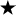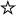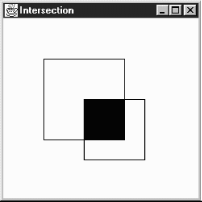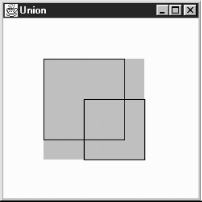home | O'Reilly's CD bookshelfs | FreeBSD | Linux | Cisco | Cisco Exam

#Chapter 2Simple Graphics## 2.5 Rectangle

The Rectangle class encapsulates x and y coordinates and width and height (Point and Dimension information) within a single object. It is often used by methods that return a rectangular boundary as a single object: for example, Polygon.getBounds(), Component.getBounds(), and Graphics.getClipBounds(). Like Point, the Rectangle class is not a visual object and does not represent a rectangle on the screen; ironically, drawRect() and fillRect() don't take Rectangle as an argument.

### Rectangle Methods

Variables

The four public variables available for Rectangle have the same names as the public instance variables of Point and Dimension. They are all accessible directly or through use of the getBounds() method.

public int x

The x coordinate of the upper left corner.

public int y

The y coordinate of the upper left corner.

public int width

The width variable represents the size of the Rectangle along the horizontal axis (left to right). Width should not be negative; however, there is nothing within the class to prevent this from happening.

public int height

The height variable represents the size of the Rectangle along the vertical axis (top to bottom). Height should not be negative; however, there is nothing within the class to prevent this from happening.

Constructors

The following seven constructors create Rectangle objects. When you create a Rectangle, you provide the location of the top left corner, along with the Rectangle's width and height. A Rectangle located at (0,0) with a width and height of 100 has its bottom right corner at (99, 99). The Point (100, 100) lies outside the Rectangle, since that would require a width and height of 101.

public Rectangle ()

This Rectangle constructor creates a Rectangle object in which x, y, width, and height are all 0.

public Rectangle (int width, int height)

This Rectangle constructor creates a Rectangle with (x, y) coordinates of (0,0) and the specified width and height. Notice that there is no Rectangle(int x, int y) constructor because that would have the same method signature as this one, and the compiler would have no means to differentiate them.

public Rectangle (int x, int y, int width, int height)

The Rectangle constructor creates a Rectangle object with an initial x coordinate of x, y coordinate of y, width of width, and height of height. Height and width should be positive, but the constructor does not check for this.

public Rectangle (Rectangle r)

This Rectangle constructor creates a Rectangle matching the original. The (x, y) coordinates are (r.x, r.y), with a width of r.width and a height of r.height.

public Rectangle (Point p, Dimension d)

This Rectangle constructor creates a Rectangle with (x, y) coordinates of (p.x, p.y), a width of d.width, and a height of d.height.

public Rectangle (Point p)

This Rectangle constructor creates a Rectangle with (x, y) coordinates of (p.x, p.y). The width and height are both zero.

public Rectangle (Dimension d)

The last Rectangle constructor creates a Rectangle with (x, y) coordinates of (0, 0). The initial Rectangle width is d.width and height is d.height.

Shaping and sizing

public Rectangle getBounds()The getBounds() method returns a copy of the original Rectangle.

public void setBounds (int x, int y, int width, int height)public void reshape (int x, int y, int width, int height)The setBounds() method changes the origin of the Rectangle to (x, y) and changes the dimensions to width by height.

reshape() is the Java 1.0 name for this method.

public void setBounds (Rectangle r)The setBounds() method changes the origin of the Rectangle to (r.x, r.y) and changes the dimensions to r.width by r.height.

public Point getLocation()The getLocation()retrieves the current origin of this rectangle as a Point.

public void setLocation (int x, int y)public void move (int x, int y)The setLocation() method changes the origin of the Rectangle to (x, y).

move() is the Java 1.0 name for this method.

public void setLocation (Point p)The setLocation() method changes the Rectangle's origin to (p.x, p.y).

public void translate (int x, int y)

The translate() method moves the Rectangle's origin by the amount (x, y). If the original Rectangle's location (r) is (3, 4) and you call r.translate (4, 5), then r's location becomes (7, 9). x and y may be negative. translate() has no effect on the Rectangle's width and height.

public Dimension getSize ()The getSize() method retrieves the current size of the rectangle as a Dimension.

public void setSize() (int width, int height)public void resize (int width, int height)The setSize() method changes the Rectangle's dimensions to width x height.

resize() is the Java 1.0 name for this method.

public void setSize() (Dimension d)The setSize() method changes the Rectangle's dimensions to d.width x d.height.

public void grow (int horizontal, int vertical)

The grow() method increases the Rectangle's dimensions by adding the amount horizontal on the left and the right and adding the amount vertical on the top and bottom. Therefore, all four of the rectangle's variables change. If the original location is (x, y), the new location will be (x-horizontal, y-vertical) (moving left and up if both values are positive); if the original size is (width, height), the new size will be (width+2*horizontal, height+2*vertical). Either horizontal or vertical can be negative to decrease the size of the Rectangle. The following code demonstrates the changes:

```import java.awt.Rectangle;
public class rect {
public static void main (String[] args) {
Rectangle r = new Rectangle (100, 100, 200, 200);
System.out.println (r);
r.grow (50, 75);
System.out.println (r);
r.grow (-25, -50);
System.out.println (r);
}
}
```

This program produces the following output:

```java.awt.Rectangle[x=100,y=100,width=200,height=200]
java.awt.Rectangle[x=50,y=25,width=300,height=350]
java.awt.Rectangle[x=75,y=75,width=250,height=250]
```

public void add (int newX, int newY)

The add() method incorporates the point (newX, newY) into the Rectangle. If this point is already in the Rectangle, there is no change. Otherwise, the size of the Rectangle increases to include (newX, newY) within itself.

This add() method incorporates the point (p.x, p.y) into the Rectangle. If this point is already in the Rectangle, there is no change. Otherwise, the size of the Rectangle increases to include (p.x, p.y) within itself.

This add() method incorporates another Rectangle r into this Rectangle. This transforms the current rectangle into the union of the two Rectangles. This method might be useful in a drawing program that lets you select multiple objects on the screen and create a rectangular area from them.

We will soon encounter a method called union() that is almost identical. add() and union() differ in that add() modifies the current Rectangle, while union() returns a new Rectangle. The resulting rectangles are identical.

Intersections

public boolean contains (int x, int y)public boolean inside (int x, int y)The contains() method determines if the point (x, y) is within this Rectangle. If so, true is returned. If not, false is returned.

inside() is the Java 1.0 name for this method.

public boolean contains (Point p)The contains() method determines if the point (p.x, p.y) is within this Rectangle. If so, true is returned. If not, false is returned.

public boolean intersects (Rectangle r)

The intersects() method checks whether Rectangle r crosses this Rectangle at any point. If it does, true is returned. If not, false is returned.

public Rectangle intersection (Rectangle r)

The intersection() method returns a new Rectangle consisting of all points that are in both the current Rectangle and Rectangle r. For example, if r = new Rectangle (50, 50, 100, 100) and r1 = new Rectangle (100, 100, 75, 75), then r.intersection (r1) is the Rectangle (100, 100, 50, 50), as shown in Figure 2.13.

public Rectangle union (Rectangle r)

The union() method combines the current Rectangle and Rectangle r to form a new Rectangle. For example, if r = new Rectangle (50, 50, 100, 100) and r1 = new Rectangle (100, 100, 75, 75), then r.union (r1) is the Rectangle (50, 50, 125, 125). The original rectangle is unchanged. Figure 2.14 demonstrates the effect of union(). Because fillRect() fills to width-1 and height-1, the rectangle drawn appears slightly smaller than you would expect. However, that's an artifact of how rectangles are drawn; the returned rectangle contains all the points within both.

#### Figure 2.13: Rectangle intersection#### Figure 2.14: Rectangle unionMiscellaneous methods

public boolean isEmpty ()

The isEmpty() method checks whether there are any points within the Rectangle. If the width and height of the Rectangle are both 0 (or less), the Rectangle is empty, and this method returns true. If either width or height is greater than zero, isEmpty() returns false. This method could be used to check the results of a call to any method that returns a Rectangle object.

public int hashCode ()

The hashCode() method returns a hash code for the rectangle. The system calls this method when a Rectangle is used as the key for a hash table.

public boolean equals (Object object)

The equals() method overrides the Object's equals() method to define what equality means for Rectangle objects. Two Rectangle objects are equal if their x, y, width, and height values are equal.

public String toString ()

The toString() method of Rectangle displays the current values of the x, y, width, and height variables. For example:

```java.awt.Rectangle[x=100,y=200,width=300,height=400]
```